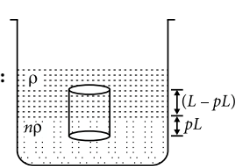Fluid statics
Question

# Two non-mixing liquids of densities $\rho$ and  are put in a container. The height of each liquid is $h$. A solid cylinder of length $L$ and density $d$ is put in this container. The cylinder floats with its axis vertical and length $pL$ in the denser liquid. The density $d$ is equal to

Moderate
Solution

##Using law of floatation, Weight of cylinder = Upthrust by two liquids$\begin{array}{l}L×A×d×g=n\rho ×\left(pL×A\right)g+\rho \left(L-pL\right)Ag\\ d=np\rho +\rho \left(1-p\right)=\left(np+1-p\right)\rho \\ d=\left\{1+\left(n-1\right)p\right\}\rho \end{array}$

Get Instant Solutions